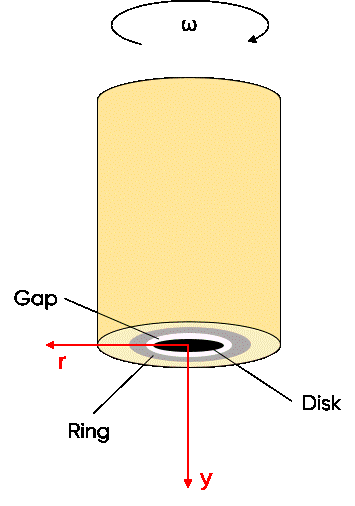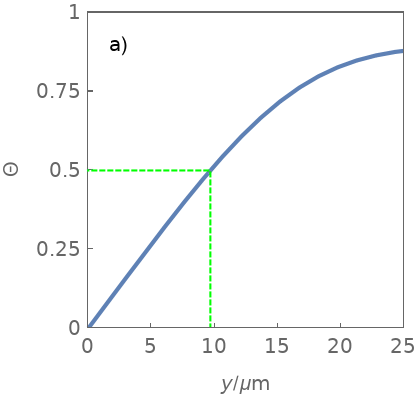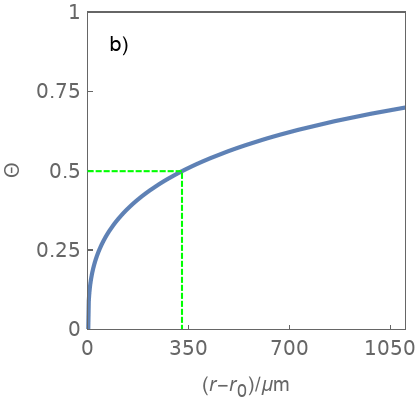Topic 5 min read# The RRDE as a pH probe in situ

Latest updated: August 9, 2023

Introduction

The Rotating Ring Disk Electrode (RRDE) ring can be used as a potentiometric or an amperometric in situ pH probe. This is extremely useful to study electrochemical reactions that involve OH or H+ ions, for example, electrodeposition and electrocatalysis. The key applications concerned by this probe include: fuel cells, depollution, sensors, hydrogen storage, metal/air batteries etc.

Theoretical background

The Rotating Disk Electrode (RDE) allows the study of electrochemical reactions in controlled steady-state hydrodynamic conditions, giving access to mass-transport and kinetic parameters [1, 2]. The addition of a ring, concentric to the disk, allows the collection of species produced at the disk (Figure 1). In some applications, theoretical discrepancies allow the identification of intermediate species and the study of bulk homogenous species reactions produced at the disk .Figure 1: Description of the rotating ring-disk electrode

Another interesting application concerns the use of the ring as a potentiometric or amperometric interfacial pH probe. Such rings have value as probes, as it is far easier to detect a pH change along the radial direction $r$ than along the normal direction $y$ (Figure 1).

Using the work carried out by Albery et al. , Hessami et al. , and the Mathematica simulation software, we were able to plot the concentration profiles of a species produced at the disk along both the normal axis $y$ (Figure 2a) and the radial axis $r$ (Figure 2b). It is assumed that there are no homogeneous reactions, that the transport of species is only by diffusion-convection, and that the disk electrode is homogeneously accessible.

The variable $\Theta$ is the normalized concentration:

$$\Theta=\frac{C-C^\mathrm{d}}{C^\mathrm{b}-C^\mathrm{d}}$$

Where $C$ is the concentration of the species, $C^\mathrm{d}$ the concentration at the disk surface and $C^\mathrm{b}$ the bulk concentration of the considered species.

$r_0$ is the disk radius. What can be seen by comparing Fig. 2a with Fig. 2b is that for a given concentration, for example, $\Theta=0.5$, the measurement probe should be placed a little below 10 µm over the disk in the normal direction (Fig. 2a), or at a little below 350 µm from the disk edge in the radial direction at $y = 0$ (Fig. 2b). Placing a ring at 350 µm from the disk is much easier than approaching a probe at 10 µm from the electrode. This is why the RRDE as a pH probe is an interesting candidate.Figure 2: Normalized concentration profile $\Theta$ as a function of
a) the normal distance $y$ at $r = 0$ and b) the radial distance from
the disk edge $r – r_0$ at $y = 0$.

Practical examples from the literature

Several papers show the use of an RRDE to monitor interfacial or surface pH changes. The seminal work by Albery et al.  presents results obtained on a bismuth-plated ring electrode. The ring is left at open-circuit while the Ni disk is polarized at various currents.

The work by Hessami et al.  shows experimental data obtained in the case of metallic electrodeposition. Indeed, metallic ions reduction occurs via hydroxylation, water hydrolysis and hydrogen evolution. Platinum black deposited on the potentiometric ring is used as a reversible material for hydrogen ions.

Iridium oxide, deposited on a Ti ring, was also used as a pH sensing material . It was used to detect pH changes during Oxygen Reduction Reaction (ORR, causing pH increase) and water reduction (causing pH decrease).

Another application includes the study of ethanol oxidation, whose reaction rate strongly depends on pH . In this particular work , they used Pt as the material for H+ ions detection on the ring, which was polarized at 0.1 V/RHE.

A Boron-Doped Diamond ring-disk electrode was used in a quiescent electrolyte to locally control and adjust the pH  at the disk. Water electrolysis and protons production occur on the ring, while Hg stripping occurs on the disk using Cyclic Voltammetry. The stripping peaks existence and position depend on the pH.

A similar method was used with a ring-disk electrode composed of an Au disk and a Pt ring electrode, under rotating condition (1600 rpm) where a constant potential is held on the Au disk to catalyze CO2 Reduction Reaction (CO2RR), while potential cycling is applied to the Pt ring electrode to detect reaction products transported from disk to the ring . The position of the peaks is pH-dependent. A different ring-disk electrode with an Iridium oxide ring was used to potentiometrically probe the local surface pH changes.

Conclusions

Because the kinetics and mass-transport conditions are well described in both cases, the rotating ring disk electrode is a method similar to an Ultra MicroElectrode (UME) probe used in the close vicinity above a second electrode (known in the world of Scanning Electrochemical Microscopy as generation/collection mode). In the particular case of pH sensing the rotating ring disk electrode shows the advantage of being less complicated to implement, as the probing electrode does not need to be in the diffusion layer thickness to be able to detect changes, but can be placed hundreds of micrometers away from the edge of the disk in the radial direction. Due to this versatility, applications are numerous, for example, electrodeposition, depollution, electrocatalysis, and the method is relatively easy to implement.

References

 W. J. Albery, E. J. Calvo, J. Chem. SoC., Faraday Trans. 1, 1983, 79, 2583

 S. Hessami, C. W. Tobias, AIChE Journal, 39, 1993, 1, 149

 P. Steegstra, E. Ahlberg, JEAC, 685, 2012, 1

 M. C. Figueiredo, R. M. Arán-Ais, V. Climent, T. Kallio, J. M. Feliu, ChemElectroChem 2015, 2, 1254

 T. L. Read, E. Bitziou, M. B. Joseph, J. V. Macpherson, Anal. Chem. 2014, 86, 367

 F. Zhang, A. C. Co, Angew. Chem. Int. Ed., 59, 2020, 4, 1674

Rotating ring disk electrode BluRev pH probe pH sensing

## Related products

Work smarter. Not harder.

Tech-tips, theory, latest functionality, new products & more.

No thanks!